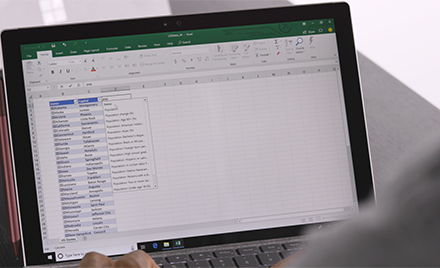# How to do percentages in Excel

Excel provides you different ways to calculate percentages. For example, you can use Excel to calculate the percentage of correct answers on a test, discount prices using various percent assumptions, or percent change between two values. Calculating a percentage in Excel is an easy two-step process. First, you format the cell to indicate the value is a percent, and then you build the percent formula in a cell.### Microsoft Excel

Turn data into insights.

Get Excel

### Format values as percentages

To show a number as a percent in Excel, you need to apply the Percentage format to the cells. Simply select the cells to format, and then click the Percent Style (%) button in the Number group on the ribbon’s Home tab. You can then increase (or decrease) the the decimical place as needed. (See Rounding issues below for more information.)In Excel, the underlying value is always stored in decimal form. So, even if you’ve used number formatting to display something as a percentage (10%), that’s just what it is—formatting, or a symbolic representation of the underlying value. Excel always performs calculations on that underlying value, which is a decimal (0.1). To double-check the underlying value, select the cell, press Ctrl + 1, and look in the Sample box on the General category.Here are a few things to keep in mind when formatting percentages:

Format existing values—When you apply percentage formatting to a cell that already has a number in it, Excel multiplies that number by 100 and adds the % sign at the end. So for example, if you type 10 into cell A2 and then apply the percentage number format, Excel will multiply your number by 100 to show it as a percentage (remember that 1% is one part of one hundred), so you’ll see 1000% displayed in the cell, not 10%. To get around this, you can calculate your numbers as percentages first.

For example, if you type the formula =10/100 in cell A2, Excel will display the result as 0.1. If you then format that decimal as a percentage, the number will be displayed as 10%, as you ‘d expect. You can also just type the number in its decimal form directly into the cell—that is, type 0.1 and then apply percentage format.

Rounding issues—Sometimes what you see in a cell (e.g., 10%) doesn’t match the number you expected to see (e.g., 9.75%). To see the true percentage in the cell, rather than a rounded version, increase the decimal places. Again, Excel always uses the underlying value to perform calculations.

Format empty cells—Excel behaves differently when you pre-format empty cells with percentage formatting and then enter numbers. Numbers equal to and larger than 1 are converted to percentages by default; numbers smaller than 1 that are not preceded with a zero are multiplied by 100 to convert them to percentages. For example, if you type 10 or .1 in a preformatted cell, you’ll see 10% appear in the cell. Now, if you type 0.1 in the cell, Excel will return 0% or 0.10% depending on the decimal setting.

Format as you type—If you type 10% directly in the cell, Excel will automatically apply percentage formatting. This is useful when you want to type just a single percentage on your worksheet, such as a tax or commission rate.

Negative percentages—If you want negative percentages to be formatted differently—for example, to appear as red text or within parentheses—you can create a custom number format such as 0.00%;[Red]-0.00% or 0.00%_);(0.00%).

### Calculating percentages

As with any formula in Excel, you need to start by typing an equal sign (=) in the cell where you want your result, followed by the rest of the formula. The basic formula for calculating a percentage is =part/total.

In the example below, Actual Points/Possible Points = Grade %:Say you want to reduce a particular amount by 25%, like when you’re trying to apply a discount. Here, the formula will be: =Price*1-Discount %. (Think of the “1” as a stand-in for 100%.)To increase the amount by 25%, simply replace the minus sign in the formula above with a plus sign.

The next example is slightly more complicated. Say the earnings for your department are \$2,342 in November and \$2,500 in December and you want to find the percentage change in earnings between these two months. To find the answer, divide the difference between December and November earnings (\$158) by the value of the November earning (\$2,342).See more examples of calculating percentages and learn more about how to format numbers as percentages. Also, start your free trial of Excel.

Editor’s note 3/7/2019:
This blog post was updated to reflect the blog’s current editorial style.

## Related posts### Bringing AI to Excel—4 new features announced today at Ignite

AI-powered features for Excel, including new data types, dynamic arrays, Ideas, and more, will help you expedite data analysis and make better decisions.### Excel with Microsoft Excel in Office 365

A twin, Jeremy, shares his top tips and tricks from The Twins Challenge: Office 365 versus Office 2019.### Office 2019 is now available for Windows and Mac

Today, we are announcing the general availability of Office 2019 for Windows and Mac. Office 2019 is the next on-premises version of Word, Excel, PowerPoint, Outlook, Project, Visio, Access, and Publisher.